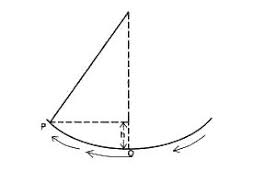# An oscillating pendulum has a velocity of 2ms-1 at the equilibrium position O and velocity at same point P. Using the diagram above, calculate the height of...

Question 1

An oscillating pendulum has a velocity of 2ms-1 at the equilibrium position O and velocity at same point P. Using the diagram above, calculate the height of P above O. (Take g = 10ms2)